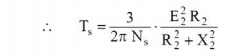Home | | Electrical Machines II | Rotor Output

# Rotor Output

If there were no copper losses in the rotor, the output would equal rotor input and the rotor would run at synchronous speed Ns.

Rotor Output

If Tg newton metre is the gross torque developed and N r.p.m is the speed of the rotor, thenIf there were no copper losses in the rotor, the output would equal rotor input and the rotor would run at synchronous speed Ns.It is clear that if the input power to rotor is "Pr" then "s.Pr" is lost as rotor Cu loss and the remaining (1 - s) Pr is converted into mechanical power. Consequently, induction motor operating at high slip has poor efficiency.Hence the approximate efficiency of an induction motot is = 1-s. Thus if the slip of an induction motor is 0.125, then its approximate efficiency is = 1 – 0.125 = 0.875 or 87.5%.

## 1. Torque Equations

The gross torque Tg developed by an induction motor is given by;## 2. Rotor Torque

The torque T developed by the rotor is directly proportional to:

(i)                rotor current

(ii)             rotor e.m.f

(iii)           power factor of the rotor circuit

µ E2I2cosϕ2

Or  T=KE2I2cosϕ2

Where

I2 = rotor current at standstill

E2 = rotor e.m.f at standstill

cosϕ2 = rotor p.f. at standstill

Note. The values o rotor e.m.., rotor current and rotor power factor are taken or the given conditions.

## 3. Starting Torque (Ts)

Let,

E2 = rotor e.m.f. per phase at standstill

X2 = rotor reactance per phase at standstill

R2 = rotor resistance per phaseGenerally, the stator supply voltage V is constant so that flux per pole  set up by the stator is also fixed. This in turn means that e.m.f. E2 induced in the rotor will be constant.Where K1 is another constant.

It is clear that magnitude of starting torque would depend upon the relative values of R2 and X2 i.e., rotor resistance/phase and standstill rotor reactance/phase.

It can shown that K = 3/2π Ns,Note that here Ns is in r.p.s.

## 4. Condition for Maximum Starting Torque

It can be proved that starting torque will be maximum when rotor resistance/phase is equal to standstill rotor reactance/phase.Hence starting torque will be maximum when:

Rotor resistance/phase = Standstill rotor reactance/phase

Under the condition of maximum starting torque,  ϕ2 = 45° and rotor power factor is 0.707 lagging.Fig. 3.14 shows the variation of starting torque with rotor resistance. As the rotor resistance is increased from a relatively low value, the starting torque increases until it becomes maximum when R2 = X2. If the rotor resistance is increased beyond this optimum value, the starting torque will decrease.

## 5. Effect of Change of Supply VoltageTherefore, the starting torque is very sensitive to changes in the value of supply voltage. For example, a drop of 10% in supply voltage will decrease the starting torque by about 20%. This could mean the motor failing to start if it cannot produce a torque greater than the load torque plus friction torque.

Study Material, Lecturing Notes, Assignment, Reference, Wiki description explanation, brief detail
Electrical machines : Three Phase Induction Motor : Rotor Output |